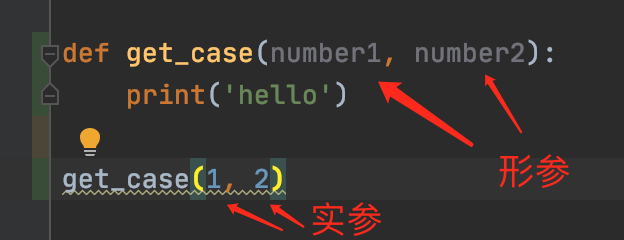### python 类各方法参数_python 各种参数类型

1.实参和形参2. 默认值参数（缺省参数）

def hahh(a,b=3,d=4):print(a,b,d)

hahh(1)

1

3

4

3. 位置参数

4. 关键字参数

defshow(n,s):for c ins:print(f'{n} — {c}’)

show(s=’hello’,n=1)

①直接按照顺序传递实参,叫做位置方式传参

②将形式参数的名字写上,按照赋值语句的格式传递,叫做关键字方式传参,并且全是关键字实参的时候,没有顺序要求.

③位置方式和关键字方式可以混用.

④混合使用的时候注意,关键字方式传参,只能写在位置方式的后面,并且顺序不能混乱.

5. 不定长位置参数

*args 在参数中定义了该形参后,那可以通过 *args 接收多个不确定个数的位置参数

def hah(*args):

i= 1

for x inargs:

i*=xprint(i)

hah(1,2,3,4)

6. 不定长关键字参数

**kwargs 在参数中定义了该形参后,那可以通过 **kwargs 接收多个不确定个数的关键字参数

def show(**kwargs):print(kwargs)

show(a=1)

show(a=1, b=2)

show(a=1, b=2, c=3)

def 函数名(*args, **kwargs):

7. 混合参数(了解)

def func(a,b,c,d,e, *args,f=1,g=2,**kwargs):